Get instant live expert help with Excel or Google Sheets“My Excelchat expert helped me in less than 20 minutes, saving me what would have been 5 hours of work!”

#### Post your problem and you’ll get Expert help in seconds.

Your message must be at least 40 characters
Our professional Expert are available now. Your privacy is guaranteed.

# How to Get the First Entry by Month and Year in Excel

To get the first entry by month and year in Excel we can use the combination of INDEX, MATCH and TEXT functions. A formula should be defined as array and dates should be sorted out in the ascending order. This step by step tutorial will assist all levels of Excel users in extracting the first value by month and year.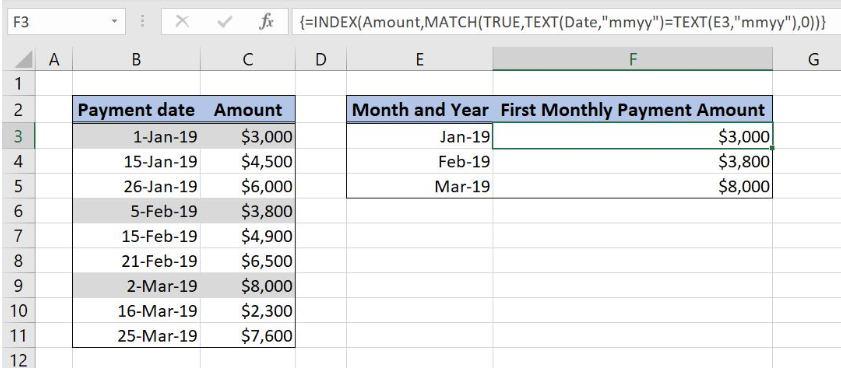Figure 1. Get the first payment amount by month and year

## Syntax of the INDEX formula

`=INDEX(array, row_num, column_num)`

The parameters of the INDEX function are:

• array – a range of cells where we want to get a data
• row_num – a number of a row in the array for which we want to get a value
• column_num – a column in the array which returns a value.

Excel INDEX function returns a value of the cell with a defined position of the cell in an array (row, column).

## Syntax of the MATCH formula

`=MATCH(lookup_value, lookup_array, [match_type])`

The parameters of the MATCH function are:

• lookup_value – a value which we want to find in the lookup_array
• lookup_array – the array where we want to find a value
• [match_type] – a type of match. We put 0 which is an exact match.

Excel MATCH function retrieves the position of the cell in an array.

## Syntax of the TEXT formula

`=TEXT(value, format_text)`

The parameters of the TEXT function are:

• value – a number that will be converted to the text format
• format_text – a format that will be used for the number

Excel TEXT function converts a number to the text in a specified format.

## Setting up Our Data to Get First Entry by Month and Year

Our first table consists of 2 columns: “Payment date” (column B) and “Amount” (column C). Payment dates are sorted out from the oldest to the newest date. The second table consists of two columns: “Month and Year” (column E) and “First Monthly Payment Amount” (column F).

Idea is to get the first monthly and yearly payment amount from the left table and to put it in column F.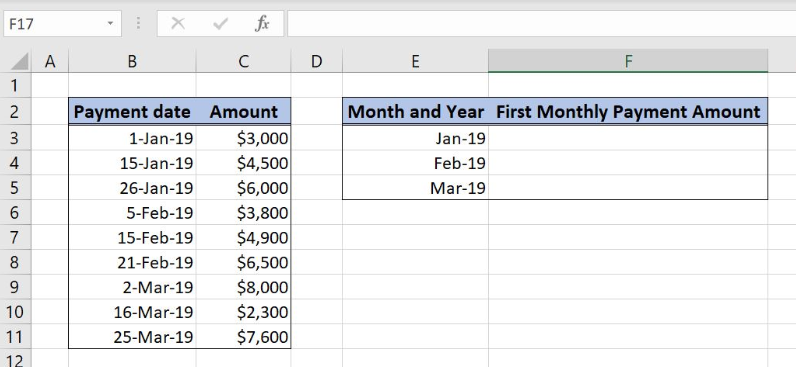Figure 2. Table structure for First Month and Year entry

## Retrieve the first payment amount entry by month and year

We want to get the first payment amount by month and the year from column C. For this we will use the combination of INDEX, MATCH and TEXT functions and define the entire formula as an array.

The formula looks like:

`{=INDEX(Amount,MATCH(TRUE,TEXT(Date,"mmyy")=TEXT(E3,"mmyy"),0))}`

Since INDEX and MATCH functions are dealing with arrays we will create a named ranges Amount and Date to make the formula more clear.

To create a named range we should follow the steps:

• Select the cell range that should be named
• Click on the name box in Excel
• Write the name for the cell range and press enter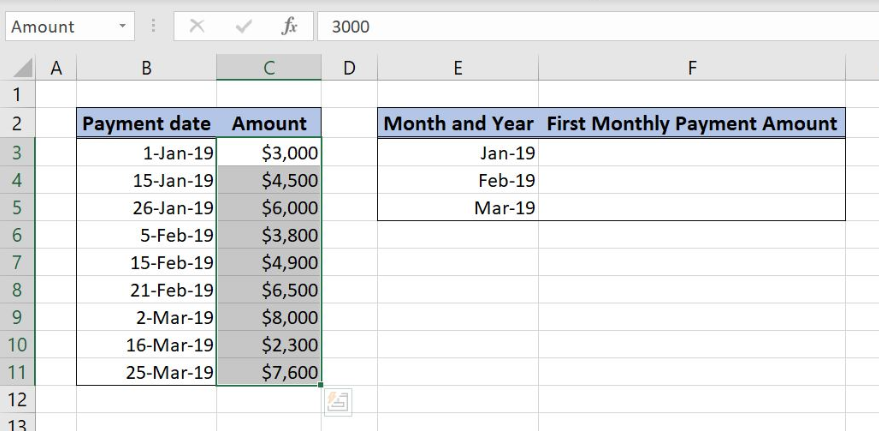Figure 3. Create a named range for column “Amount”

Named range Amount refers to the cell range C3:C1, and named range Date to the cell range B3:B11.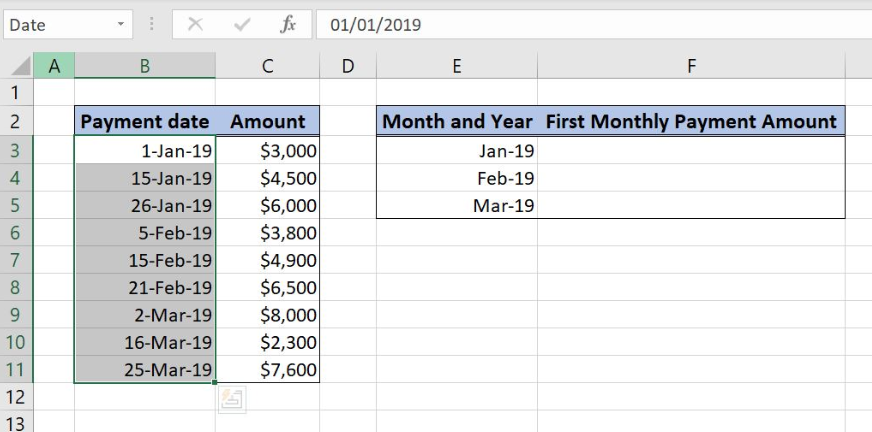Figure 4. Create a named range for column “Payment date”

Now we can explain formula syntax in details:

`{=INDEX(Amount,MATCH(TRUE,TEXT(Date,"mmyy")=TEXT(E3,"mmyy"),0))}`

For INDEX function array is the named range Amount because we want to get the data from the Amount column.

Row_num is the other formula part:

`MATCH(TRUE,TEXT(Date,"mmyy")=TEXT(E3,"mmyy"),0)`

With MATCH function we are searching for the first date by month and year in the named range Date that is matchable with the month and year in the column E. TEXT function is used to convert date format to “mmyy” format.

After TEXT function Date named range will look like:

`{“0119”;“0119”;“0119”;“0219”;“0219”;“0219”;“0319”;“0319”;“0319”}`

Values are then compared with a value from the cell E3, “0119”. TEXT function converted the cell value in the format “mmyy”. The result is an array of TRUE and FALSE values:

`{TRUE;TRUE;TRUE;FALSE;FALSE;FALSE;FALSE;FALSE;FALSE}.`

MATCH function returns the number 1 as a result since value TRUE is the first exact match in the output array. Number 1 goes into the INDEX function as the row number in the column Amount and formula output in cell F3 is \$3,000:

`=INDEX(Amount,1)`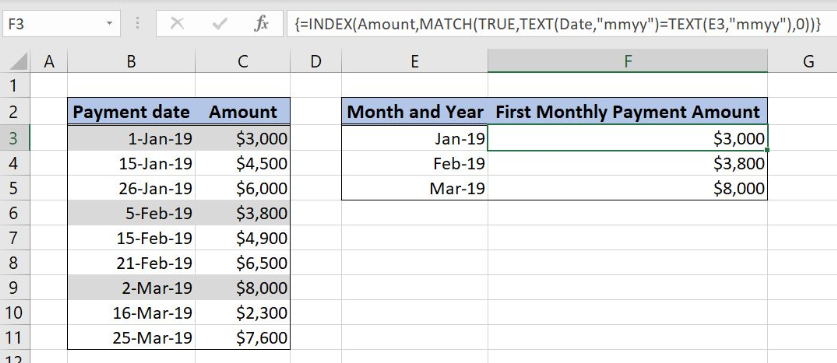Figure 5. Get the first entry by month and year using INDEX, MATCH and TEXT functions

To apply the function, we need to follow these steps:

• Select cell F3 and click on it
• Insert the formula: `=INDEX(Amount,MATCH(TRUE,TEXT(Date,"mmyy")=TEXT(E3,"mmyy"),0))`
• A formula has to be converted to array formula by clicking ctrl+shift+enter
• Drag the formula down to the other cells in the column by clicking and dragging the little “+” icon at the bottom-right of the cell.

Most of the time, the problem you will need to solve will be more complex than a simple application of a formula or function. If you want to save hours of research and frustration, try our live Excelchat service! Our Excel Experts are available 24/7 to answer any Excel question you may have. We guarantee a connection within 30 seconds and a customized solution within 20 minutes.

### Did this post not answer your question? Get a solution from connecting with the expert.Another blog reader asked this question today on Excelchat:
Solution examplesI need a formula to compare the data in two columns and then export the mismatched data in the 3rd column
Solved by S. Q. in 20 minsUse the Vlookup Function to complete the "employee" column of table 2. Use "job Id" from table 2 as your lookup_value(s) and table 1 as your reference.
Solved by C. H. in 16 minsIf a cell in another sheet is populated I need a vlookup done. If the cell is not populated I need the cell to return blank.
Solved by T. D. in 60 minsI am trying to make a chart that turns a week range red if nothing is entered in the range. If something is entered then I would like it to turn green. Please Help
Solved by E. U. in 43 minsI need a check box to show/hide an answer of an if function
Solved by Z. U. in 23 mins## Subscribe to Excelchat.coAnother blog reader asked this question today on Excelchat: